﻿ 海卓帕斯冷却水除垢防垢

#400-800-4341

昆山旺铨科技冷却水处理

旺诠科技 （昆山）有限公司是台资电子类上市企业， 政府对其总排水量有限制。为了减少工厂的冷却水循环系统的排水量和用药量，提高生产设备的运行效率，工厂采用了海卓帕斯电子除垢仪 。

工厂共有四套冷却水循环系统：一套为空压机冷却系统，另外三套为冷冻机冷却系统：该四套系统的主管径分别为：DN150和DN250，现场实际安装了4台P160和2台PU10的海卓帕斯电子除垢仪，以及旁滤设备（袋式过滤器）用以过滤污垢和藻类等杂质。

附：系统示意图客户现场实拍：空压机冷却系统安装图片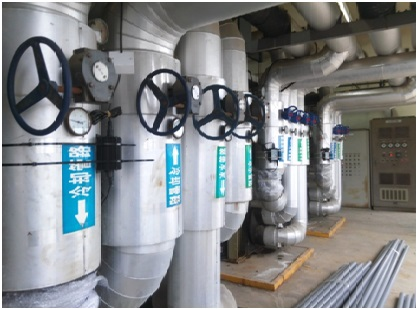冷冻机冷却系统安装图片

从8月8日安装之日起，旺铨公司停止使用化学药剂，对该冷却水系统的水质连续监测三个月，检测结果如下：

 编号 检测指标 自来水11/24 水样实测数值 08/08 08/29 10/10 11/24 备注 1 冷却塔水总硬度 120 #1 >>500#2 >>500#3 = 245#4 = 165 #1 = 520#2 = 205#3 = 270#4 = 215 #1 = 130#2 = 260#3 = 155#4 = 195 #1 = 190#2 = 停机#3 = 380#4 = 450 2 PH 7.8 #1 = 8.8#2 = 8.9#3 = 8.0#4 = 8.4 #1 = 8.7#2 = 8.6#3 = 8.6#4 = 8.6 #1 = 8.5#2 = 8.7#3 = 8.3#4 = 8.7 #1 = 8.6#2 = 停机#3 = 8.8#4 = 8.7 3 TDS 300 #1 = 2600#2 = 2200#3 = 600#4 = 500 #1 = 1200#2 = 600#3 = 700#4 = 800 #1 = 400#2 = 1100#3 = 400#4 = 680 #1 = 700#2 = 停机#3 = 2300#4 = 1800 4 电导率 390 #1 = 1170#2 = 620#3 = 760#4 = 790 #1 = 440#2 = 1070#3 = 480#4 = 680 #1 = 770#2 = 停机#3 = 1980#4 = 1620 5 碱度 75 #1 = 480#2 = 480#3 = 110#4 = 110 #1 = 270#2 = 135#3 = 195#4 = 180 #1 = 115#2 = 330#3 = 125#4 = 190 #1 = 165#2 = 停机#3 = 380#4 = 370 6 硫酸盐 49 #1 = 155#2 = 停机#3 = 185#4 = 195 7 氯盐 31 #1 = 63#2 = 停机#3 = 360#4 = 360 * 浓缩倍数 #1 = 2倍#2 = 停机#3 = 12#4 = 12 以氯盐为参考指标

说明1.8月8日检测时#4冷却水塔处于停工状态。当天安装了海卓帕斯设备。

说明2.11月24日#2塔停止运行，塔内无水。 #1塔待机状态，塔内有水。

说明3.10月下旬起，工厂补水大幅减少，只有少量溢流排水。

数据分析：

1.通常电导率数值比TDS大10%，因为水中有胶体物质，而TDS无法检测出胶体物质。但从上表的数据测试中，我们发现TDS比电导率大，是由于海卓帕斯帮助促成水中颗粒及胶体物质絮凝抱团，从而被过滤器拦截掉。

2.因氯盐的溶解度极高，并几乎不受外界条件影响，所以把它作为浓缩倍数的参照指标更具科学性。氯盐的浓缩倍数目前达到了12倍。而冷凝器的端差温度一直保持在3℃，整个系统运行非常平稳

3.总硬度和硫酸盐的浓缩倍数都小于4，表明海卓帕斯设备促使了离子抱团结晶析出，被袋式过滤器拦截掉。

4.冷却塔底部没有看到水藻，填料处老水垢脱落大半，同时看到塔内水质清澈，底部无泥，表明海卓帕斯设备除垢和灭藻杀菌的功效。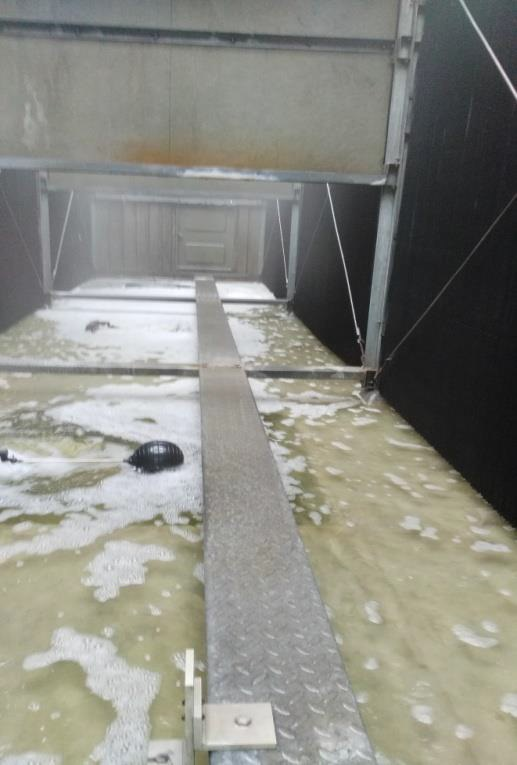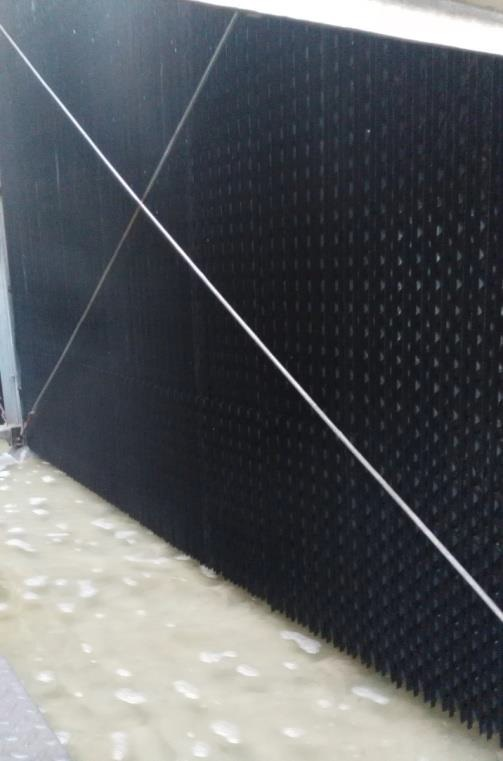结论：

1.冷却水的浓缩倍数即使达到12倍，其他各项指标依旧表现良好，在国家规范以内。制冷机端差温度始终保持在3℃以内，整个冷却水循环系统运行高效平稳。

2.使用海卓帕斯设备后，工厂补水及排水量大幅减少，实现了节能减排。

3.在化学药剂零添加的情况下，冷却塔内的藻类几乎没有，水质清澈。同时，降低了TDS等指标，变相地扩大了适用的水质条件。使用海卓帕斯水处理器后，有效地帮助了该企业实现节能减排任务，节省了管理成本。

4.使用海卓帕斯水处理器后，大幅减少化学药剂的使用，减少药剂对设备的损伤，延长生产设备的使用寿命和维修周期。

经过三个多月的持续跟踪，现确定了海卓帕斯水处理器对除垢防垢、灭藻杀菌以及物理絮凝的作用。旺诠科技（昆山）有限公司对海卓帕斯设备在冷却水循环系统的应用效果非常满意。该企业愿意提供技改交流研讨。

#### 共有-条评论【我要评论】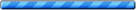我要评论: 内 容： （内容最多500个汉字，1000个字符） 验证码： 看不清？！ 1.尊重网上道德，遵守中华人民共和国的各项有关法律法规，不发表攻击性言论。 2.承担一切因您的行为而直接或间接导致的民事或刑事法律责任。 3.案例留言板管理人员有权保留或删除其管辖留言中的任意内容。
海卓帕斯案例中心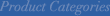#### 余热锅炉除垢防垢

联系海卓帕斯·中国
全国咨询热线：
400-800-4341

公司电话：021-67890912

企业邮箱：info@hydropathchina.com

公司地址：上海市普陀区金沙江路2145号B座10楼